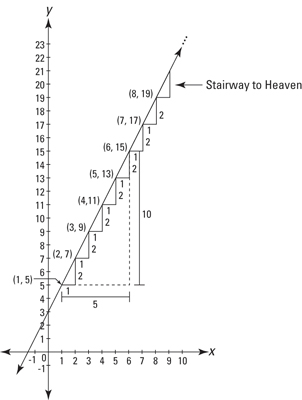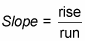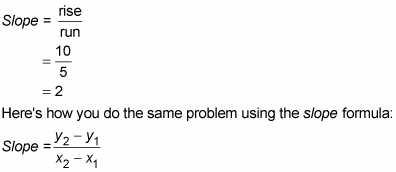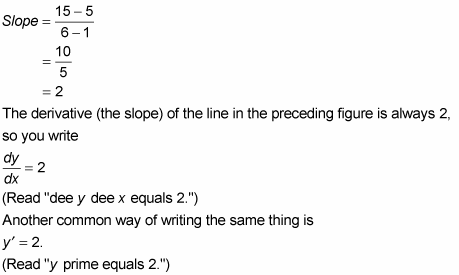##### Differential Equations For DummiesThe derivative is just a fancy calculus term for a simple idea that you probably know from algebra — slope. Slope is the fancy algebra term for steepness. And steepness is the fancy word for . . . No! Steepness is the ordinary word you’ve known since you were a kid, as in, “Hey, this road sure is steep.” Everything you study in differential calculus all relates back to the simple idea of steepness.

Here’s a little vocabulary for you: differential calculus is the branch of calculus concerning finding derivatives; and the process of finding derivatives is called differentiation. Notice that the first and third terms are similar but don’t look like the term derivative. The link between derivative and the other two words is based on the formal definition of the derivative, which is based on the difference quotient. Now you can go and impress your friends with this little etymological nugget.

Don’t be among the legions of people who mix up the slopes of horizontal and vertical lines. How steep is a flat, horizontal road? Not steep at all, of course. Zero steepness. So, a horizontal line has a slope of zero. What’s it like to drive up a vertical road? You can’t do it. And you can’t get the slope of a vertical line — it doesn’t exist, or, as mathematicians say, it’s undefined.

To find points on the line y = 2x + 3 (shown in the figure below), just plug numbers into x and calculate y: plug 1 into x and y equals 5, which gives you the point located at (1, 5); plug 4 into x and y equals 11, giving you the point (4, 11); and so on.You should remember thatThe rise is the distance you go up (the vertical part of a stair step), and the run is the distance you go across (the horizontal part of a step). Now, take any two points on the line — say, (1, 5) and (6, 15) — and figure the rise and the run. You rise up 10 from (1, 5) to (6, 15) because 15 – 5 = 10. And you run across 5 from (1, 5) to (6, 15) because 6 – 1 = 5.

Next, you divide to get the slope:You can just plug in the points (1, 5) and (6, 15):